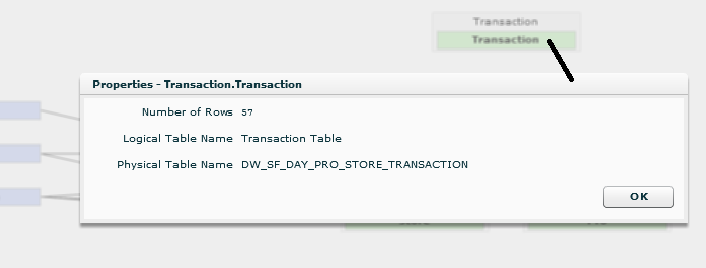How to Create Custom Week Start Day in BirstThere are organizations that work on multiple countries and follow the individual country based calendars. In that case, defining the week (Start Day and End Day) is an important requirement from a reporting perspective.

Example:

In India, the commonly followed week definition is Monday to Sunday (Monday being the start of the week). In Middle East, the commonly followed week definition is Saturday to Friday (Saturday being the start of the week).

How to Achieve:

One way to achieve this would be to upload a custom calendar into Birst stating Monday as start day of the week. The other way to achieve this is a bit tweak on the Birst Time Dimension (in-built) using custom expressions. In this post, I explain the tweak on the time dimension.

Details:

I have created a filter named “Weekstart” in Dashboard and based on filter selection the default week start day can be assigned.

In Dashboard page create a list filter for week start day as shown and name the filter as “weekstart”.In designer, just drag the measures which you need to Analyze based on Day of Week and drag the Day column from Time Dimension. Then create an expression as custom week start day as shown below:``````IIF(GetPromptValue('weekstart')=2,
IIF([Time.Day of Week]=1,Integer(7),Integer([Time.Day of Week])-1),
IIF(GetPromptValue('weekstart')=3,
IIF([Time.Day of Week]<3,Integer([Time.Day of Week])+5,Integer([Time.Day of Week])-2),
IIF(GetPromptValue('weekstart')=4,
IIF([Time.Day of Week]<4,Integer([Time.Day of Week])+4,Integer([Time.Day of Week])-3),
IIF(GetPromptValue('weekstart')=5,
IIF([Time.Day of Week]<5,Integer([Time.Day of Week])+3,Integer([Time.Day of Week])-4),
IIF(GetPromptValue('weekstart')=6,
IIF([Time.Day of Week]<6,Integer([Time.Day of Week])+2,Integer([Time.Day of Week])-5),
IIF(GetPromptValue('weekstart')=7,
IIF([Time.Day of Week]<7,Integer([Time.Day of Week])+1,Integer([Time.Day of Week])-6),
IIF(GetPromptValue('weekstart')=1,
Integer([Time.Day of Week]),'false')))))))
``````

Here I have used [Time.Day of Week] column from time dimension and altered the day numbers from 1 to 7 based on user’s week start day selection.

Below is the sample report which shows ‘Quantity of Items Sold’ based on Day from Saturday to Sunday.